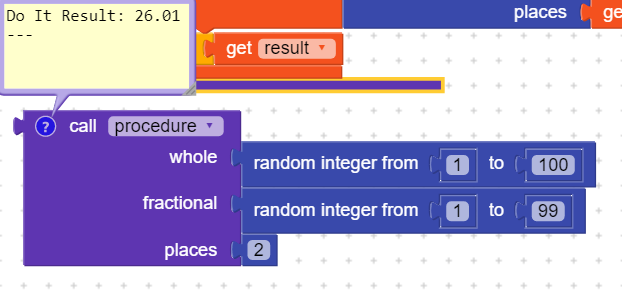# How to generate random decimal number

I can generate Random number with decimal places but but but
the decimal places numbers are not changed (as random) [as shown in below 2nd image]

Output is :

so Question is: How do I get randomize decimal places???

1 Like

You need a bit more trickery if you want decimals 0.01 - 0.09…1 Like

Just Give
me the solution1 Like

Actually I want to

1. Get random number with random 2 decimal places (like 12.45)
2. then show on display from 0 to got random number (0 to 12.45)
how can we do this ??/

Use above procedure

Please elaborate more, what do you mean by that ?

1 Like

Now if you want to show all number from 0 to random by … use

sample video is given below try to play and understand what i am looking for

Example: If I got random number 7.68 then
I want to show from 0 to 7.68 in increasing order (exactly as shown in above video)

Getting it ??